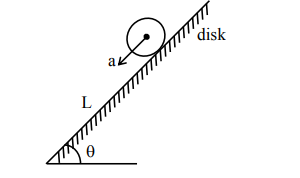# A circular disc reaches from top to bottom of an inclined

Question:

A circular disc reaches from top to bottom of an inclined plane of length ' $L$ '. When it slips down the plane, it takes time ' $t_{1}$ '. When it rolls down the

plane, it takes time $t_{2}$. The value of $\frac{t_{2}}{t_{1}}$ is $\sqrt{\frac{3}{x}}$. The

value of $x$ will be___________.

Solution:If disk slips on inclined plane, then it's acceleration

$a_{1}=g \sin \theta$

$\mathrm{L}=\frac{1}{2} \mathrm{a}_{1} \mathrm{t}_{1}^{2}$

$\Rightarrow t_{1}=\sqrt{\frac{2 L}{a_{1}}}$ $\ldots .$ (i)

If disk rolls on inclined plane, its acceleration,

$\mathrm{a}_{2}=\frac{\mathrm{g} \sin \theta}{1+\frac{\mathrm{I}}{\mathrm{mR}^{2}}}$

$a_{2}=\frac{g \sin \theta}{1+\frac{m R^{2}}{2 m R^{2}}}$

$a_{2}=\frac{2}{3} g \sin \theta$

Now $L=\frac{1}{2} \mathrm{a}_{2} \cdot \mathrm{t}_{2}^{2}$

$\Rightarrow t_{2}=\sqrt{\frac{2 L}{a_{2}}}$ $\ldots$ (ii)

Now $\frac{t_{2}}{t_{1}}=\sqrt{\frac{a_{1}}{a_{2}}}=\sqrt{\frac{3}{2}}$

$\Rightarrow x=2$